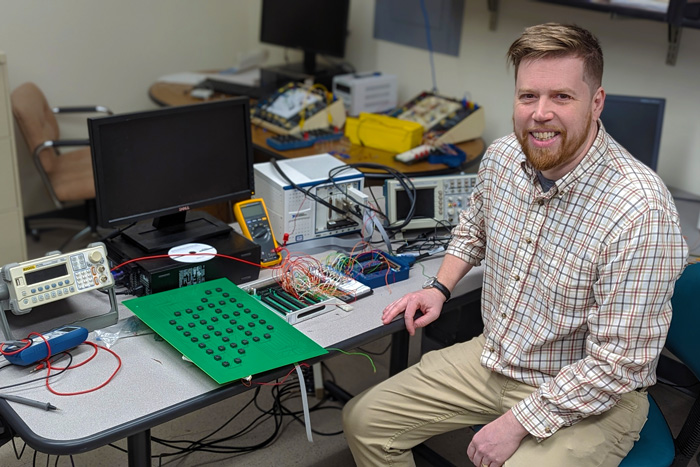# Building a Machine to Solve the 'Traveling Salesman' and Similar ProblemsProfessor of Physics Lars English and his "parallel computing machine," a small version constructed as a proof of principle. Photo by Tony Moore

## Working with a group from Cambridge University, Professor of Physics Lars English publishes new research in 'Nature' journal 'Communication Physics'

Professor of Physics Lars English, working with a theoretical group at Cambridge University, recently published a paper, "An Ising Machine Based on Networks of Subharmonic Electrical Resonators," on the construction of a "parallel computing machine" that can churn out answers to some combinatorial optimization problems. (The most famous of this sort of problem is the classic "traveling salesman" problem, detailed below.)

Such problems are notoriously difficult to solve using conventional computers and algorithms, but in building a machine to work on it, the research, published in the Nature journal Communication Physics, straddles both physics and computer science.

### To best understand it, read how English sums it up in his own words:

We built a small version of such a machine here at Dickinson, just as a proof of principle. For something like that to solve real problems (that people would be interested in), it would need to be scaled up in size. But it's a start.

The classic "combinatorial optimization problem" is the so-called "traveling salesman problem": put down N locations on a map that the salesman has to visit, then figure out in what order he should visit them so as to minimize the total traveling distance. It's the kind of problem UPS must solve all the time.

The problem is that if N gets large, the number of possible routes blows up—it goes like N-factorial, so it goes up faster than exponentially. This means that the brute-force method of testing each possibility is not feasible. There are other conventional algorithms, but they all suffer from the problem that they take a very long time when N gets large. This is why these types of problems are labeled "hard" in computer science. The immediate problem that we were trying to solve is called the max-cut problem (in computer science). Solving the max-cut problem, in turn, can help optimize the design of computer chips, where billions of transistors need to be connected efficiently.

Now, you've heard of "quantum computation," where the idea is that you are utilizing the laws of quantum mechanics themselves to perform some useful "computation" (like factoring huge numbers to break the RSA encryption scheme). But it turns out that you can also use other laws of physics, not just quantum laws. Here, we appropriated the physical laws of electricity to do something useful.

The idea is that we connect N electrical oscillators up to one another in a network of our choosing. (The network properties encode the problem we are trying to solve.) The physics will then take over and dynamically evolve this network of resonators into a final state. This final state encapsulates the optimized solution to the problem. So the physics itself found the solution organically. That's the idea.

### TAKE THE NEXT STEPS

Published January 18, 2023